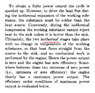# Carnot cycle - Zero Power Extremes

Joshua Pham
Hey guys,
I ran into this paper talking about the Maximum power you can obtain from a Carnot cycle: http://aapt.scitation.org/doi/abs/10.1119/1.10023 From what I understood, there are two extremes. To achieve maximum efficiency you have to make sure that the temperature of the system is never more than infinitesmally out of thermal equilibrium with its thermal reservoirs. So during the isothermal expansion, you have heat transfer coming in albeit at a very slow rate because of the negligible temperature difference between the hot reservoir and the system. It then isentropically expands to its low temperature which is infinitesmally higher than the cold reservoir and you get heat transfer out.

The other extreme is when you set the system temperature so that it does not change during the isothermal processes. From the paper this leads to all of the heat going straight from the hot sink to the cold sink and 0 power output. I don't quite understand this bit. Why does all of the heat go straight from the hot to cold sink?

Mentor
Hey guys,
I ran into this paper talking about the Maximum power you can obtain from a Carnot cycle: http://aapt.scitation.org/doi/abs/10.1119/1.10023 From what I understood, there are two extremes. To achieve maximum efficiency you have to make sure that the temperature of the system is never more than infinitesmally out of thermal equilibrium with its thermal reservoirs. So during the isothermal expansion, you have heat transfer coming in albeit at a very slow rate because of the negligible temperature difference between the hot reservoir and the system. It then isentropically expands to its low temperature which is infinitesmally higher than the cold reservoir and you get heat transfer out.

The other extreme is when you set the system temperature so that it does not change during the isothermal processes. From the paper this leads to all of the heat going straight from the hot sink to the cold sink and 0 power output. I don't quite understand this bit. Why does all of the heat go straight from the hot to cold sink?
Did you mean "during the isentropic process" rather than "during the isothermal process" in the last paragraph?

Joshua Pham
Did you mean "during the isentropic process" rather than "during the isothermal process" in the last paragraph?

No the paper says isothermal processes. I'm still trying to decipher what this means.

#### Attachments

•Capture.PNG
33.6 KB · Views: 393
Mentor
The author is talking about carrying out the cycle irreversibly, so that there is a finite temperature difference between the working fluid and the hot reservoir, and an equal finite temperature difference between the working fluid and the cold reservoir (so that, in both isothermal steps of the cycle, the working fluid is half way between the hot reservoir and the cold reservoir temperature). Under these circumstances, the heat transferred from the hot reservoir to the working fluid is equal to the heat transferred from the working fluid to the cold reservoir. So, no net work is done, and the net effect is a transfer of heat from the hot reservoir to the cold reservoir.

Joshua Pham
The author is talking about carrying out the cycle irreversibly, so that there is a finite temperature difference between the working fluid and the hot reservoir, and an equal finite temperature difference between the working fluid and the cold reservoir (so that, in both isothermal steps of the cycle, the working fluid is half way between the hot reservoir and the cold reservoir temperature). Under these circumstances, the heat transferred from the hot reservoir to the working fluid is equal to the heat transferred from the working fluid to the cold reservoir. So, no net work is done, and the net effect is a transfer of heat from the hot reservoir to the cold reservoir.

Thanks Chestermiller! My lecturer actually drew a picture like this when he attempted to explain it. Where THC and TLC are the high and low temperatures of the system respectively. "To maximise the heat transfer rate in, let THC = TLC. But then Qin = Qout." I don't really understand this statement and I'm still struggling to understand what the paper is saying#### Attachments

Mentor
The heat transfer rate into the working fluid during the expansion is ##k(T_H-T_{HC})## where k is a constant of proportionality; the heat transfer rate out of the working fluid during the compression is ##k(T_{LC}-T_C)##. If ##T_{HC}=T_{LC}=\frac{(T_H+T_C)}{2}##, then ##Q_H=Q_C## and ##W=Q_H-Q_C=0##

•Joshua Pham
Joshua Pham
The heat transfer rate into the working fluid during the expansion is ##k(T_H-T_{HC})## where k is a constant of proportionality; the heat transfer rate out of the working fluid during the compression is ##k(T_{LC}-T_C)##. If ##T_{HC}=T_{LC}=\frac{(T_H+T_C)}{2}##, then ##Q_H=Q_C## and ##W=Q_H-Q_C=0##

Starting to become clearer. How did you obtain ##T_{LC} = \frac{(T_H + T_C)}{2}## though?

Mentor
Starting to become clearer. How did you obtain ##T_{LC} = \frac{(T_H + T_C)}{2}## though?
I just assumed the expansion and compression temperatures are the same, and half way between the hot and cold reservoir temperatures, for illustrative purposes.

•Joshua Pham